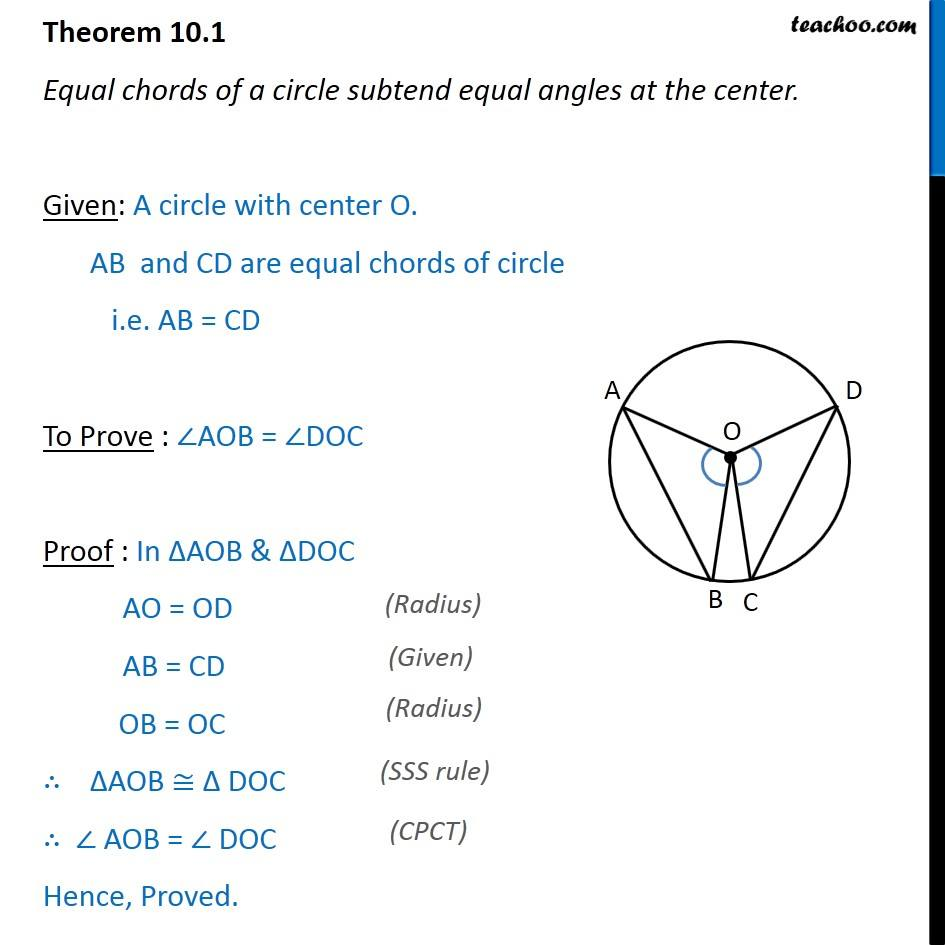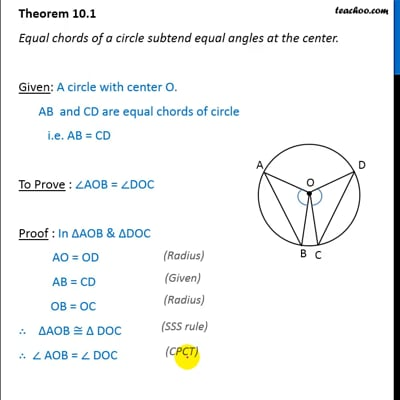Theorems

Chapter 10 Class 9 Circles (Term 2)
Serial order wiseThis video is only available for Teachoo black users

### Transcript

Theorem 10.1 Equal chords of a circle subtend equal angles at the center. Given: A circle with center O. AB and CD are equal chords of circle i.e. AB = CD To Prove : ∠AOB = ∠DOC Proof : In ΔAOB & ΔDOC AO = OD AB = CD OB = OC ∴ ∆AOB ≅ ∆ DOC ∴ ∠ AOB = ∠ DOC Hence, Proved.